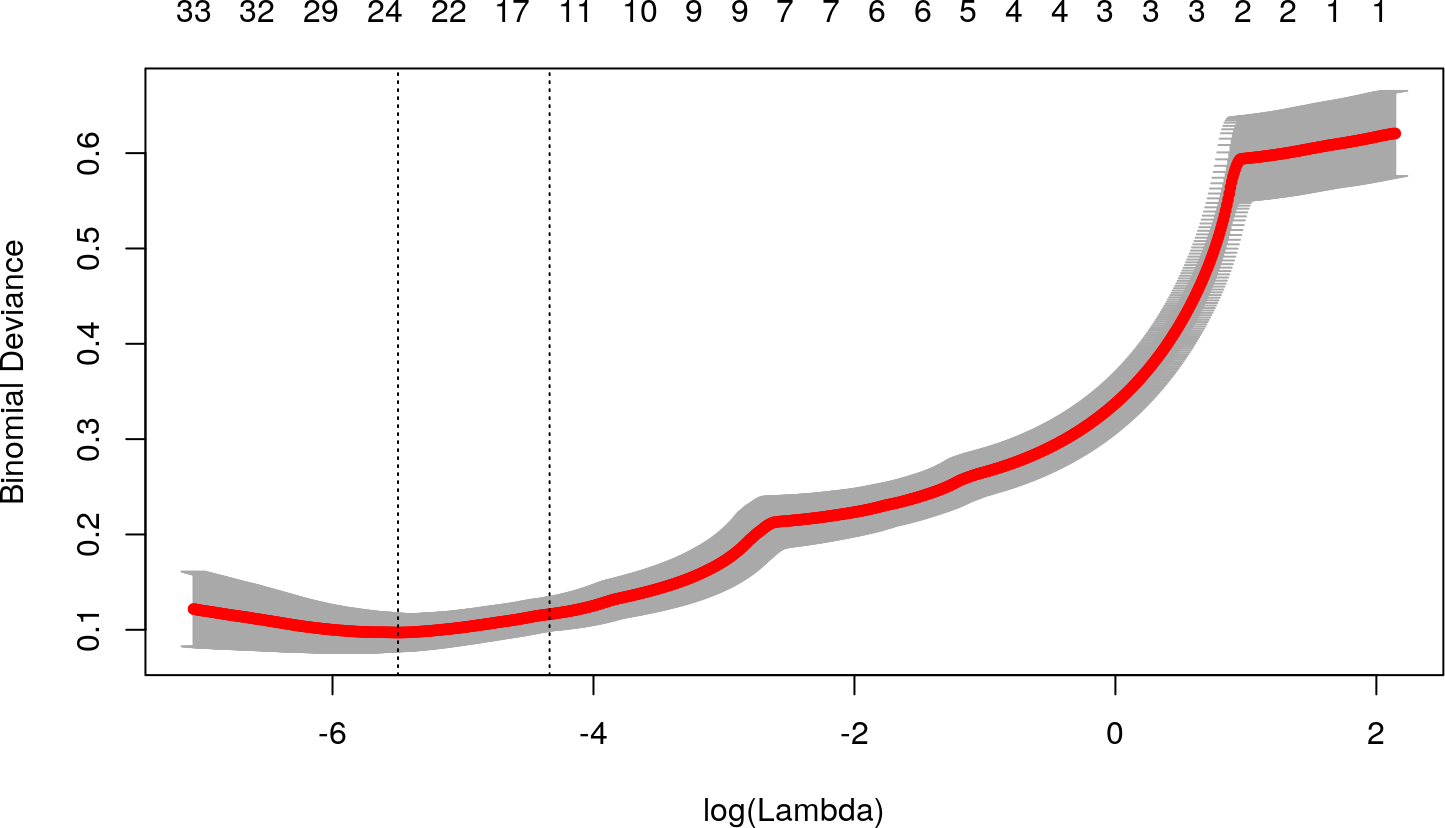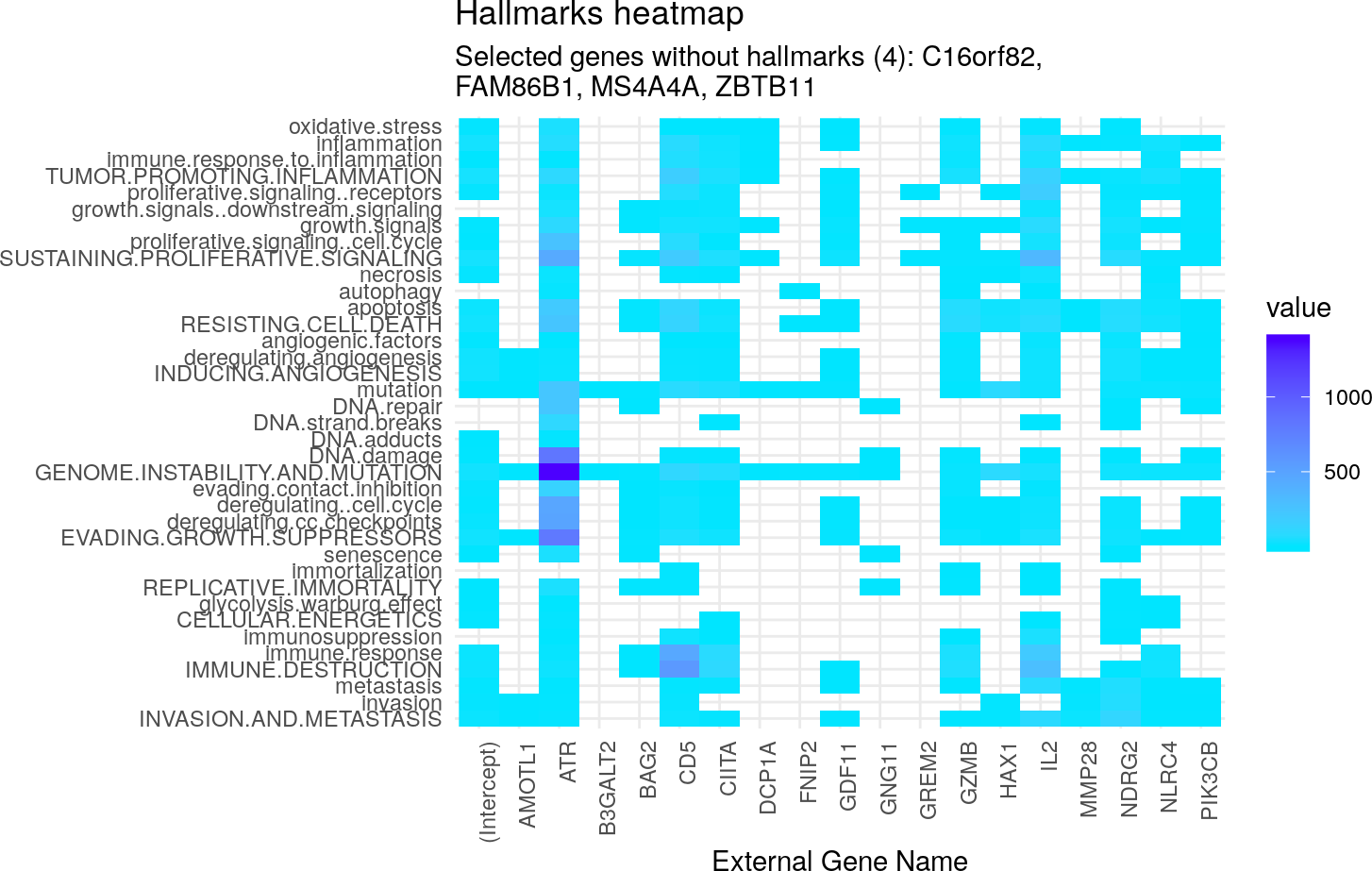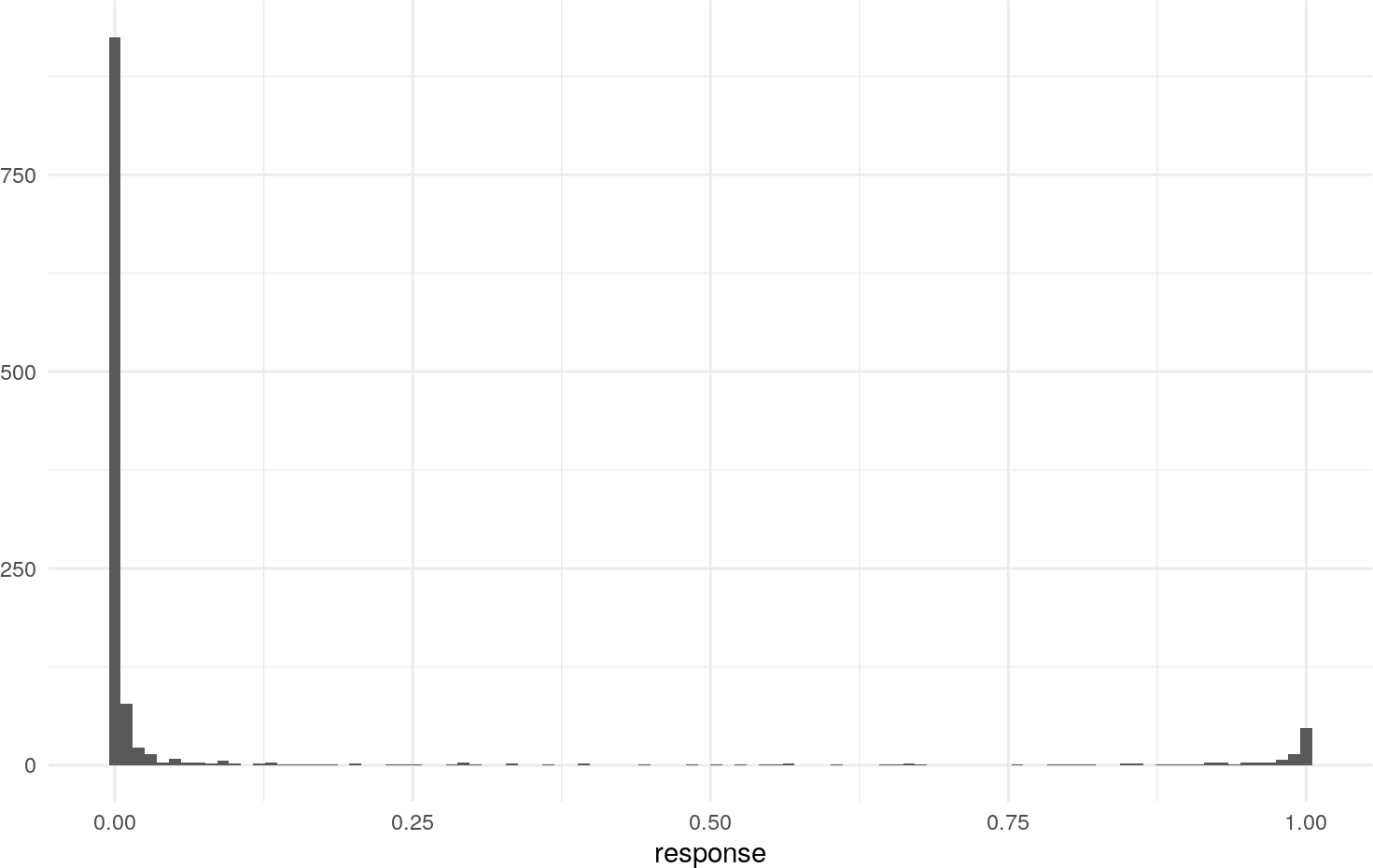# Contents

## 0.1 Instalation

``````if (!require("BiocManager"))
install.packages("BiocManager")
BiocManager::install("glmSparseNet")``````

# 1 Required Packages

``````library(dplyr)
library(ggplot2)
library(survival)
library(loose.rock)
library(futile.logger)
library(TCGAutils)
#
library(glmSparseNet)
#
# Some general options for futile.logger the debugging package
.Last.value <- flog.layout(layout.format('[~l] ~m'))
.Last.value <- loose.rock::show.message(FALSE)
# Setting ggplot2 default theme as minimal
theme_set(ggplot2::theme_minimal())``````

The data is loaded from an online curated dataset downloaded from TCGA using `curatedTCGAData` bioconductor package and processed.

To accelerate the process we use a very reduced dataset down to 107 variables only (genes), which is stored as a data object in this package. However, the procedure to obtain the data manually is described in the following chunk.

``brca <- curatedTCGAData(diseaseCode = "BRCA", assays = "RNASeq2GeneNorm", FALSE)``
``````brca <- TCGAutils::splitAssays(brca, c('01','11'))
xdata.raw <- t(cbind(assay(brca[]), assay(brca[])))

# Get matches between survival and assay data
class.v        <- TCGAbiospec(rownames(xdata.raw))\$sample_definition %>% factor
names(class.v) <- rownames(xdata.raw)

# keep features with standard deviation > 0
xdata.raw <- xdata.raw %>%
{ (apply(., 2, sd) != 0) } %>%
{ xdata.raw[, .] } %>%
scale

set.seed(params\$seed)
small.subset <- c('CD5', 'CSF2RB', 'HSF1', 'IRGC', 'LRRC37A6P', 'NEUROG2',
'NLRC4', 'PDE11A', 'PIK3CB', 'QARS', 'RPGRIP1L', 'SDC1',
'TMEM31', 'YME1L1', 'ZBTB11',
sample(colnames(xdata.raw), 100))

xdata <- xdata.raw[, small.subset[small.subset %in% colnames(xdata.raw)]]
ydata <- class.v``````

# 3 Fit models

Fit model model penalizing by the hubs using the cross-validation function by `cv.glmHub`.

``````fitted <- cv.glmHub(xdata, ydata,
family  = 'binomial',
network = 'correlation',
nlambda = 1000,
network.options = networkOptions(cutoff = .6,
min.degree = .2))``````

# 4 Results of Cross Validation

Shows the results of `1000` different parameters used to find the optimal value in 10-fold cross-validation. The two vertical dotted lines represent the best model and a model with less variables selected (genes), but within a standard error distance from the best.

``plot(fitted)``## 4.1 Coefficients of selected model from Cross-Validation

Taking the best model described by `lambda.min`

``````coefs.v <- coef(fitted, s = 'lambda.min')[,1] %>% { .[. != 0]}
coefs.v %>% {
data.frame(ensembl.id  = names(.),
gene.name   = geneNames(names(.))\$external_gene_name,
coefficient = .,
stringsAsFactors = FALSE)
} %>%
arrange(gene.name) %>%
knitr::kable()``````
ensembl.id gene.name coefficient
(Intercept) (Intercept) -6.8189811
CD5 AMOTL1 -1.1200445
NLRC4 ATR -1.4434577
PIK3CB B3GALT2 -0.3880002
ZBTB11 BAG2 -0.3325728
ATR C16orf82 1.2498303
IL2 CD5 0.6327083
GDF11 CIITA -0.2676642
DCP1A DCP1A 0.2994599
AMOTL1 FAM86B1 0.4430643
BAG2 FNIP2 -0.1841676
C16orf82 GDF11 0.0396368
FAM86B1 GNG11 0.2025462
FNIP2 GREM2 0.6101758
MS4A4A GZMB 1.1614778
B3GALT2 HAX1 -0.0867011
GNG11 IL2 3.0659065
NDRG2 MMP28 1.1142519
HAX1 MS4A4A -0.1516836
GREM2 NDRG2 -0.2014884
CIITA NLRC4 0.4256103
GZMB PIK3CB -2.7663573
MMP28 ZBTB11 -0.8438023

## 4.2 Hallmarks of Cancer

``geneNames(names(coefs.v)) %>% { hallmarks(.\$external_gene_name)\$heatmap }``
``## Cache found``## 4.3 Accuracy

``## [INFO] Misclassified (11)``
``## [INFO]   * False primary solid tumour: 7``
``## [INFO]   * False normal              : 4``

Histogram of predicted responseROC curve

``## Setting levels: control = Primary Solid Tumor, case = Solid Tissue Normal``
``## Setting direction: controls < cases``